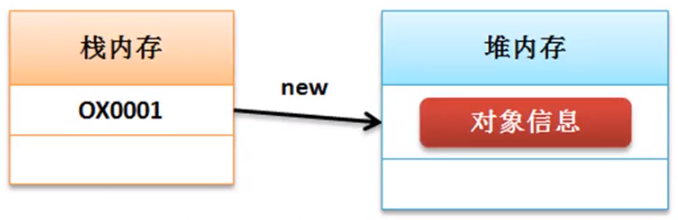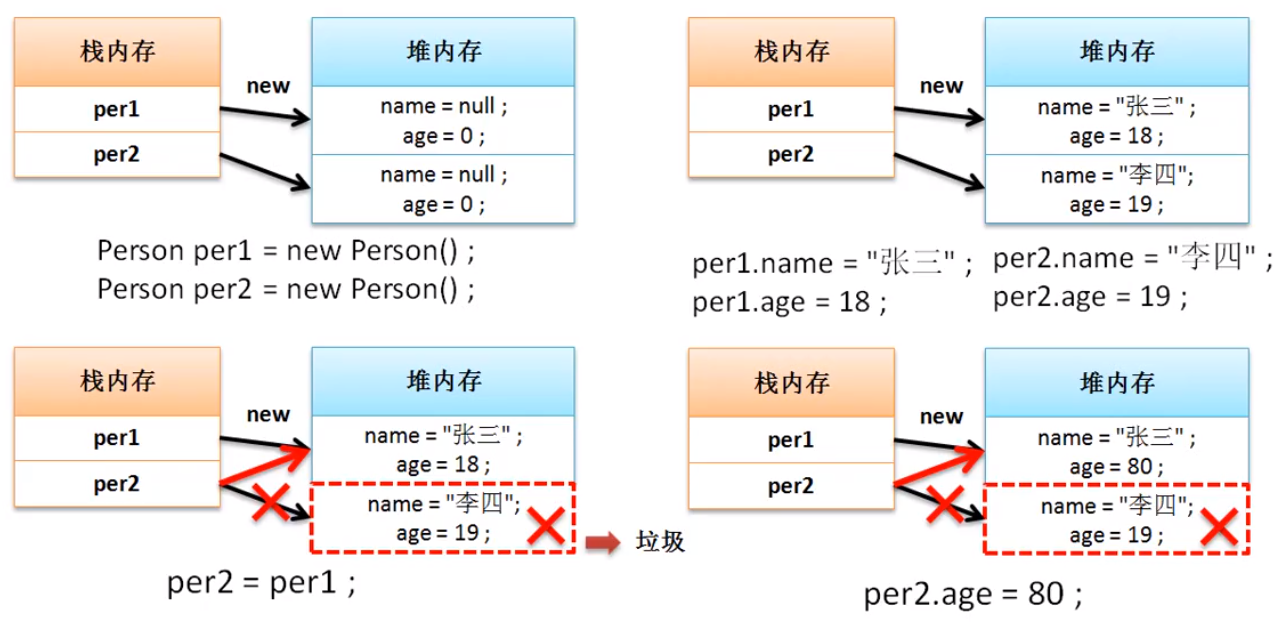# 面向对象简介

Java语言最大的特点在于面向对象的编程设计.

• 封装性(内部操作对外部而言不可见)
• 继承性(在已有结构的基础上进行功能的扩充)
• 多态性(在继承性的基础上扩充而来的概率,指的是类型的转换处理)

# 类与对象简介

类是一个模板,对象是类可以使用的实例

• 成员属性:有时简称属性
• 操作方法:定义对象具有的处理行为

# 类与对象的定义及使用

### 案例代码-定义一个类

``````package Class;

class Person { // 定义一个类
String name; // 人员的姓名
int age; // 人员的年龄

public void tell() {
System.out.println("姓名:" + name + "年龄" + age);
}
}

public class Demo {
public static void main(String[] args) {}
}
``````

• 声明并实例化对象:类名称 对象名称=new 类名称();
• 分步完成:
• 声明对象:类名称 对象名称=null
• 实例化对象:对象名称 =new 类名称()

## 案例代码-使用对象

``````package Class;

class Person { // 定义一个类
String name; // 人员的姓名
int age; // 人员的年龄

public void tell() {
System.out.println("姓名:" + name + " " + "年龄" + age);
}
}

public class Demo {
public static void main(String[] args) {
Person per = new Person(); // 声明并实例化对象
per.name = "张三";
per.age = 30;
per.tell(); // 调用方法

Person per2 = null; // 声明对象
per2 = new Person(); // 实例化对象
per.name = "李四";
per.age = 40;
per.tell(); // 调用方法
}
}
``````

# 对象内存分析

## 堆栈内存

```两块最常用的内存空间
+ 堆内存:保存的是对象的基本信息
+ 栈内存:保存的是一块堆内存的地址,通过地址找到堆内存,然后找到对象```## 内存分析# 对象引用分析

``````package Class;

public class YinYongDemo {
public static void main(String[] args) {
Person per = new Person(); // 声明并实例化对象
per.name = "张三";
per.age = 30;
Person per2 = per;
per2.age = 25;
per.tell(); // 调用方法
}
}
``````
``````package Class;

public class YingYongDemo2 {
public static void main(String[] args) {
Person per = new Person(); // 声明并实例化对象
per.name = "张三";
per.age = 30;
change(per); // 等价于 Person temp=per;
per.tell(); // 调用方法
}

public static void change(Person temp) {
temp.age = 80;
}
}
``````

# 引用与垃圾产生分析

``````package Class;

public class Rubbish {
public static void main(String[] args) {
Person per1 = new Person(); // 声明并实例化对象
Person per2 = new Person();
per1.name = "张三";
per1.age = 18;
per2.name = "李四";
per2.age = 19;
per2 = per1; // 引用传递
per2.age = 80;
per1.tell(); // 进行方法调用
}
}
``````